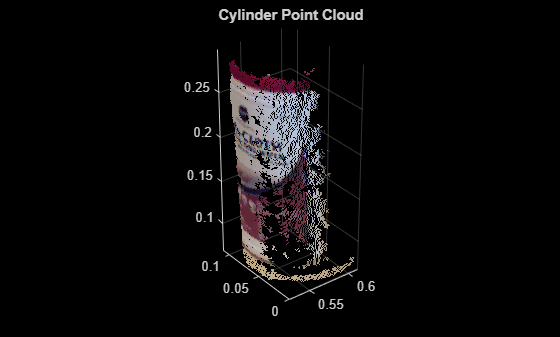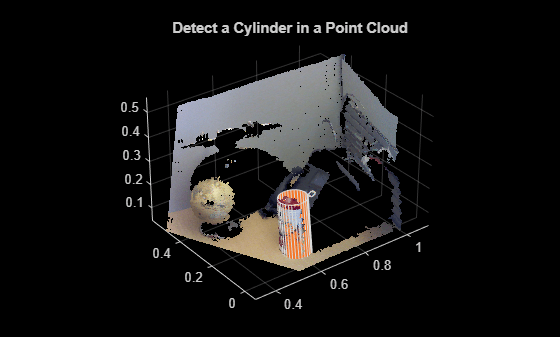# pcfitcylinder

Fit cylinder to 3-D point cloud

## Syntax

``model = pcfitcylinder(ptCloudIn,maxDistance)``
``model = pcfitcylinder(ptCloudIn,maxDistance,referenceVector)``
``model = pcfitcylinder(ptCloudIn,maxDistance,referenceVector,maxAngularDistance)``
``````[model,inlierIndices,outlierIndices] = pcfitcylinder(ptCloudIn,maxDistance)``````
``````[___,meanError] = pcfitcylinder(ptCloudIn,maxDistance)``````
``[___] = pcfitcylinder(___,Name,Value)``

## Description

````model = pcfitcylinder(ptCloudIn,maxDistance)` fits a cylinder to a point cloud with a maximum allowable distance from an inlier point to the cylinder. This function uses the M-estimator SAmple Consensus (MSAC) algorithm to find the cylinder.```
````model = pcfitcylinder(ptCloudIn,maxDistance,referenceVector)` fits a cylinder to the point cloud with additional orientation constraints specified by the 1-by-3 reference orientation input vector.```
````model = pcfitcylinder(ptCloudIn,maxDistance,referenceVector,maxAngularDistance)` additionally specifies the maximum allowed absolute angular distance.```
``````[model,inlierIndices,outlierIndices] = pcfitcylinder(ptCloudIn,maxDistance)``` additionally returns linear indices to the inlier and outlier points in the point cloud input.```
``````[___,meanError] = pcfitcylinder(ptCloudIn,maxDistance)``` additionally returns the mean error of the distance of the inlier points to the model.```

example

````[___] = pcfitcylinder(___,Name,Value)` uses additional options specified by one or more `Name,Value` pair arguments.```

## Examples

collapse all

`load("object3d.mat");`

Display the point cloud.

```figure pcshow(ptCloud) xlabel("X(m)") ylabel("Y(m)") zlabel("Z(m)") title("Original Point Cloud")```Set the maximum point-to-cylinder distance (5 mm) for cylinder fitting.

`maxDistance = 0.005;`

Set the region of interest to constrain the search.

```roi = [0.4,0.6,-inf,0.2,0.1,inf]; sampleIndices = findPointsInROI(ptCloud,roi);```

Set the orientation constraint.

`referenceVector = [0,0,1];`

Detect the cylinder and extract it from the point cloud by specifying the inlier points.

```[model,inlierIndices] = pcfitcylinder(ptCloud,maxDistance,... referenceVector,SampleIndices=sampleIndices); pc = select(ptCloud,inlierIndices);```

Plot the extracted cylinder.

```figure pcshow(pc) title("Cylinder Point Cloud")```Load a MAT file containing a point cloud into the workspace.

`load("object3d.mat");`

Display the point cloud.

```figure pcshow(ptCloud) xlabel("X(m)") ylabel("Y(m)") zlabel("Z(m)") title("Detect a Cylinder in a Point Cloud")```

Set the maximum point-to-cylinder distance for cylinder fitting to 5mm.

`maxDistance = 0.005;`

Specify a region of interest (ROI) to constrain the fitting function.

```roi = [0.4 0.6; -inf 0.2; 0.1 inf]; sampleIndices = findPointsInROI(ptCloud,roi);```

Set the orientation constraint for the fitting function

`referenceVector = [0 0 1];`

Detect the cylinder in the specified ROI of the point cloud and extract it.

```model = pcfitcylinder(ptCloud,maxDistance,referenceVector, ... SampleIndices=sampleIndices);```

Plot the model of the detected cylinder.

```hold on plot(model)```## Input Arguments

collapse all

Point cloud, specified as a `pointCloud` object. If the `Normal` property of the input `ptCloud` is empty, the function populates it with values to meet the requirements of the fitting algorithm.

Maximum distance from an inlier point to the cylinder, specified as a scalar value. Specify the distance in units that are consistent with the units you are using for the point cloud.

Data Types: `single` | `double`

Reference orientation, specified as a 1-by-3 vector.

Maximum absolute angular distance, specified as a scalar value. The maximum angular distance is measured in degrees between the direction of the fitted cylinder and the reference orientation.

### Name-Value Arguments

Specify optional pairs of arguments as `Name1=Value1,...,NameN=ValueN`, where `Name` is the argument name and `Value` is the corresponding value. Name-value arguments must appear after other arguments, but the order of the pairs does not matter.

Before R2021a, use commas to separate each name and value, and enclose `Name` in quotes.

Example: `'SampleIndices'`,`[]`.

Linear indices of points to sample in the input point cloud, specified as the comma-separated pair consisting of '`SampleIndices`' and a column vector. An empty vector means that all points are candidates to sample when fitting the cylinder during the RANSAC iteration. If you specify a subset of points, the function fits the model by sampling only those points in the subset. Providing a subset of points can significantly speed up the process by reducing the number of trials. You can generate the indices vector using the `findPointsInROI` method of the `pointCloud` object.

Maximum number of random trials for finding inliers, specified as the comma-separated pair consisting of '`MaxNumTrials`' and a positive integer. To improve robustness of the output, increase this value. However, doing so adds additional computations.

Percentage for finding maximum number of inliers, specified as the comma-separated pair consisting of '`Confidence`' and a numeric scalar, in the range (0 100). To improve the robustness of the output, increase this value. However, doing so adds additional computations.

## Output Arguments

collapse all

Geometric model of cylinder, returned as a `cylinderModel` object.

The coefficients for the output model are set to zero when:

• The input point cloud does not contain enough valid points.

• The algorithm cannot find enough inlier points.

Linear indices of the inlier points in the input point cloud, returned as a column vector.

Linear indices of the outlier points in the input point cloud returned as a column vector.

Mean error of the distance of inlier points to the model, returned as a scalar value.

## Algorithms

The function returns a geometric model that describes the cylinder. This function uses the M-estimator SAmple Consensus (MSAC) algorithm to find the cylinder. The MSAC algorithm is a variant of the RANdom SAmple Consensus (RANSAC) algorithm.

The fitting algorithm for the `pcfitcylinder` function requires point cloud normals. Therefore, if the `Normal` property for the input point cloud is empty, the function fills it. When the function fills the `Normal` property, it uses six points to fit the local cylinder. If six points do not work and the fitting fails, consider calling the `pcnormals` function which enables you to select the number of points to use.

 Torr, P. H. S., and A. Zisserman. “MLESAC: A New Robust Estimator with Application to Estimating Image Geometry.” Computer Vision and Image Understanding. Volume 78, Issue 1, April 2000, pp. 138-156.